﻿ Java String类的性质与比较 - 自由资讯

# Java String类的性质与比较

##### 目录
• 1.什么是String？
• 2.String类的构造方法
• 3.String类的性质
• 4.String之间的相互比较

## 1.什么是String？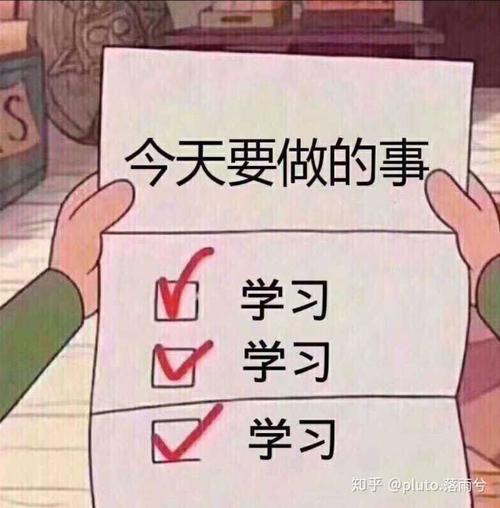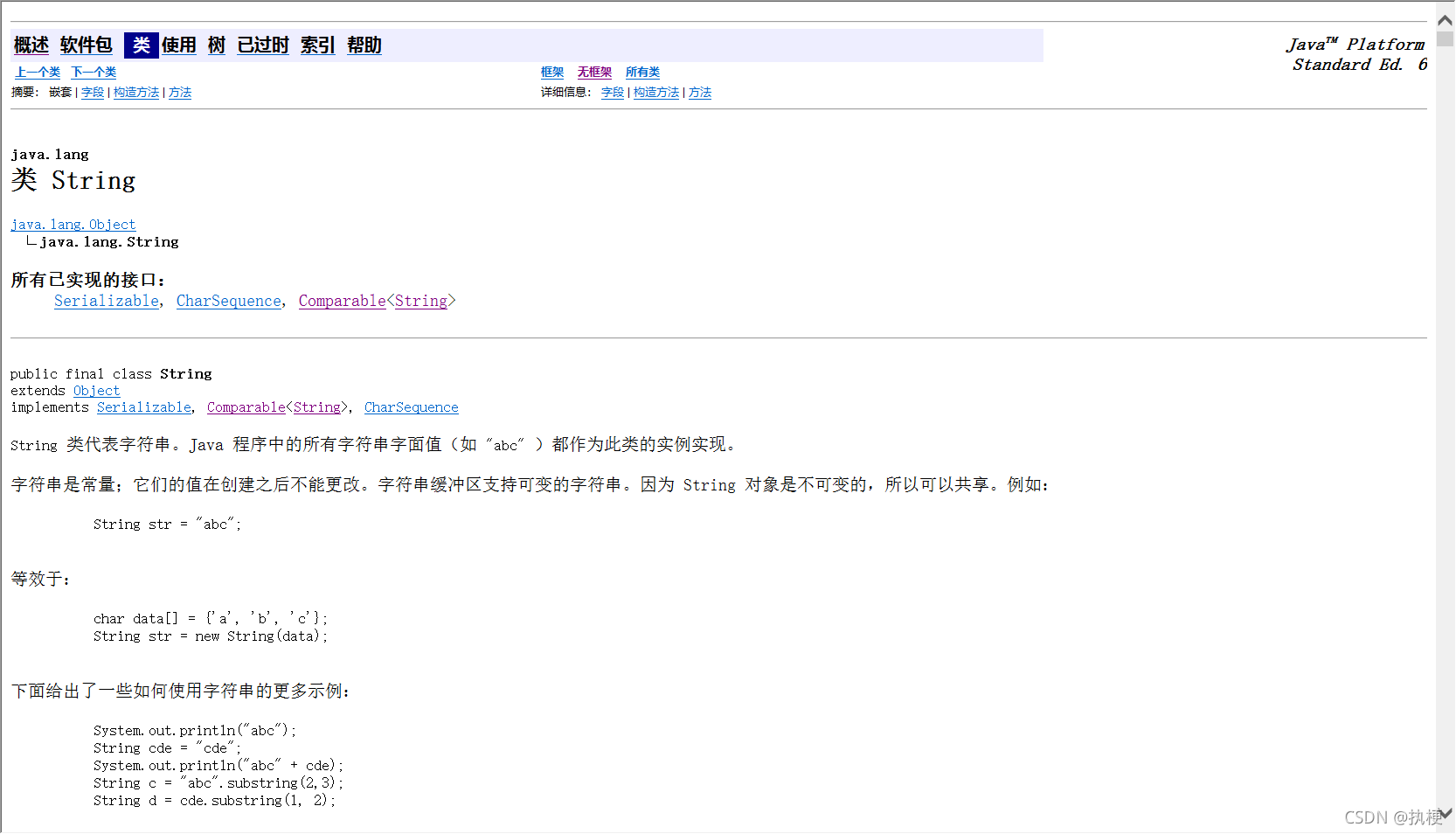## 2.String类的构造方法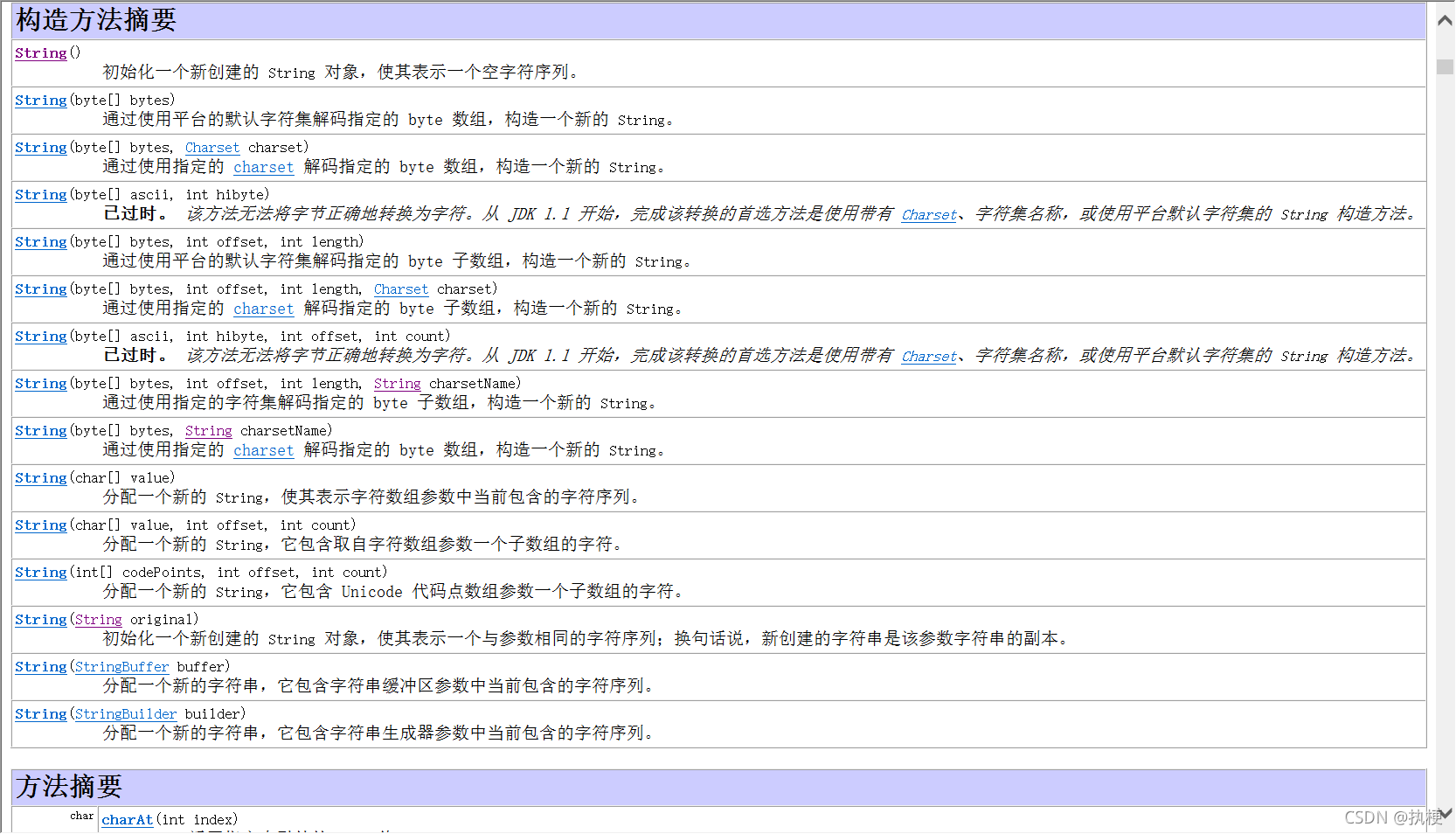1.`public String(char[] value)`

`public String(char[] value)`

```public class myString {
public static void main(String[] args) {
char[] arr={'a','b','c','d'};
String string = new String(arr);
System.out.println(string);//abcd

}
}```

2.`public String(char[] value, int offset, int count)`

`public String(char[] value,int offset,int count)`

```public class myString {
public static void main(String[] args) {
char[] arr={'a','b','c','d','e','f'};
String string = new String(arr,1,4);
System.out.println(string);//bcde
//offect为开始复制的下标，count为复制的长度

}
}```

byte类型的数组转化为String其实也同理与char，这里也不过多叙述，我们使用String时通常是像常用数据类型一样直接使用给它赋值，学好String最主要还是学好它的性质。## 3.String类的性质

jdk文档中可以很清楚的看见在String类的声明中它被finaly修饰，所以它具有不可变性。

```public class myString {
public static void main(String[] args) {
int b=100;
System.out.println(b);//100
b=10;
System.out.println(b);//10

String a="aaaa";
System.out.println(a);//aaaa
a="bbbb";
System.out.println(a);bbbb

}
}```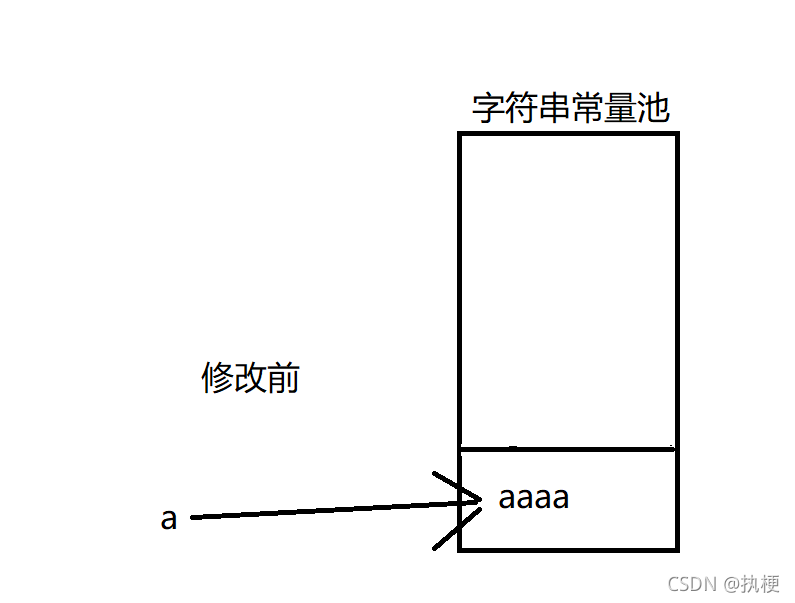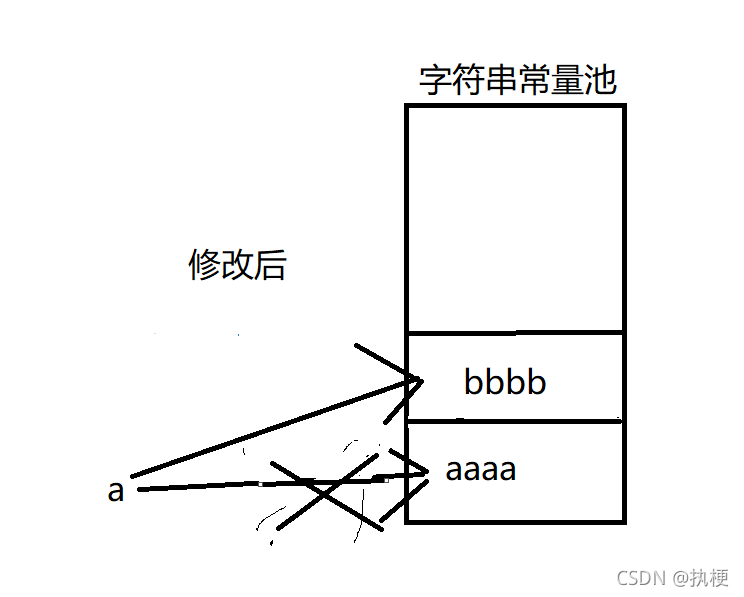## 4.String之间的相互比较

String类是非常重要且常见的类，我们使用的场景非常多，对String类之间是否相等的比较情况场景非常多见，掌握比较String比较的能力必不可少。

1.

```
public class myString {
public static void main(String[] args) {

String a1=new String("AAAA");
String a2=new String("BBBB");
System.out.println(a1==a2);//false
System.out.println(a2.equals(a2));//true
}
}```

2.

``` public class myString {
public static void main(String[] args) {

String a1=new String("AAAA");
String a2=a1;
System.out.println(a1==a2);//true
System.out.println(a2.equals(a2));//true
}
}

public static void main(String[] args) {

String a1=new String("AAAA");
String a2=new String(a1);
System.out.println(a1==a2);//false
System.out.println(a2.equals(a2));//true
}
}```

3、

```public class myString {
public static void main(String[] args) {

String a1="aaaa";
String a2="aaaa";
System.out.println(a1==a2);//true
System.out.println(a2.equals(a2));//true
}
}```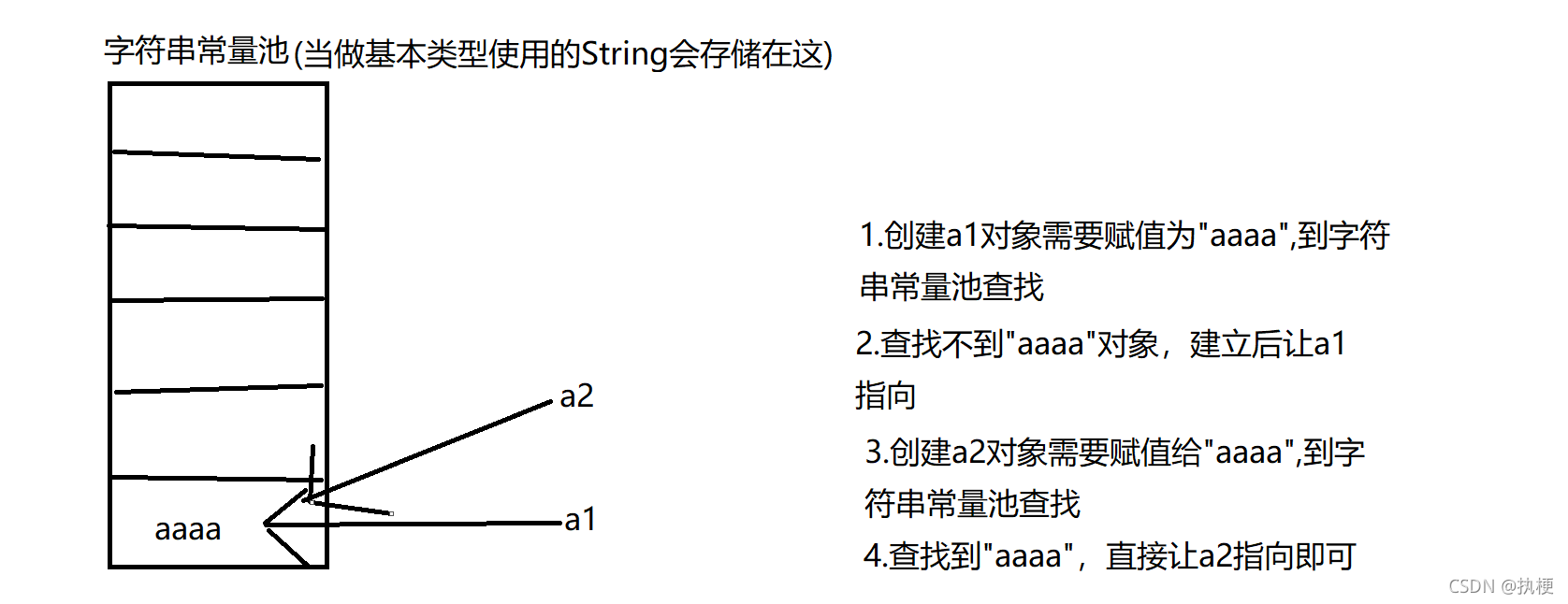﻿